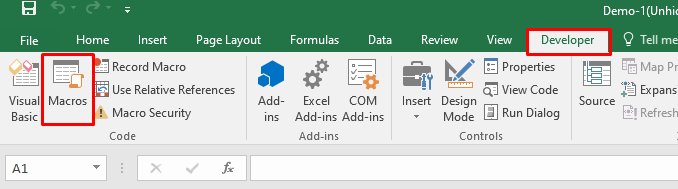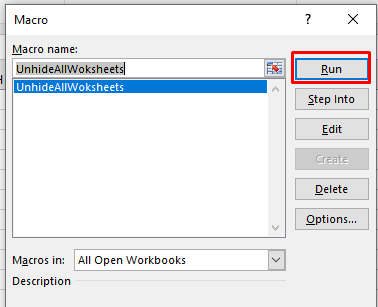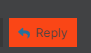# Basic concept of Macros

Hello Everyone, Hope you are doing good

I would like to present this post who are looking for the expressions related to Macro

# How to Run the Macro

`````` Here are the steps to run the macros
``````
• Go to the Developer tab
• Click on Macros
• In the Macro dialog box, select the macro you want to run.
• Click on Run button.# Unhide All Worksheets Except the Active Sheet

User needs to unhide all the workbook at one go, try to execute macros.

``````Sub UnhideAllWoksheets()
Dim ws As Worksheet
For Each ws In ActiveWorkbook.Worksheets
ws.Visible = xlSheetVisible
Next ws
End Sub
``````

# Insert A Row in the workbook

Insert a blank row after every row in the selected range, try to execute macros.

``````Option Explicit
Sub InsertAlternateRows()
Dim rng As Range
Dim CountRow As Integer
Dim i As Integer
Set rng = Selection
CountRow = rng.EntireRow.Count
For i = 1 To CountRow
ActiveCell.EntireRow.Insert
ActiveCell.Offset(2, 0).Select
Next i
End Sub
``````

# Sum A Workbook Excel Column

In this code to Add the values in the columns, Try with Macros

``````Sub SumColumns()

Dim Nb_Rows As Integer
Nb_Rows = 4

With ThisWorkbook.Worksheets("Sheet2")
.Cells(1, 3).FormulaR1C1 = "=Sum(RC1, RC2)"
.Cells(1, 3).AutoFill Destination:=.Range(.Cells(1, 3), .Cells(Nb_Rows, 3))
.Range(.Cells(1, 3), .Cells(Nb_Rows, 3)) = .Range(.Cells(1, 3), .Cells(Nb_Rows, 3)).Value
.Cells(Nb_Rows + 1, 3).FormulaR1C1 = "=SUM(R1C:R[-1]C)"
End With

End Sub
``````

# Sort Workbook and Performing the condition

In this Code it will sort in alphabetically order and it will perform the if condition

``````Option Explicit
Public log As Variant
Sub SortSheetsTabName()
Application.ScreenUpdating = False
Dim ShCount As Integer, i As Integer, j As Integer, k As Integer
Dim Ws As Worksheet
Dim Range As Range
Dim Bool As Boolean
Set Ws = ActiveWorkbook.Worksheets(1)
Ws.Activate
With Ws
On Error Resume Next
Set Range = .Range("B1:B20")
Range.FillDown
log = Err.Number & ". " & Err.Description & vbCrLf
log = log & "Error Range"
Debug.Print log
Range.Select
End With
On Error GoTo 0
ShCount = Sheets.Count
For i = 1 To ShCount - 1
For j = i + 1 To ShCount
log = log & "Iteration: " & j & vbCrLf
If Sheets(j).Name < Sheets(i).Name Then
Sheets(j).Move before:=Sheets(i)
End If
Next j
Next i
k = 0
Application.ScreenUpdating = True
Set Ws = Nothing
Set Range = Nothing
End Sub
Function Message()
Call SortSheetsTabName
Message = log
End Function
``````
Workflow_SS

Hope this will be helpful# Questions

For questions on your retrieval case open a new topic and get individual support

# Feedback

Clickbutton to share your thoughts

Regards
Gokul

8 Likes

Looks awesome - great job @Gokul001!

1 Like